# RSA Prepared by SITI ZAINAH ADNAN If you

• Slides: 36RSA Prepared by: SITI ZAINAH ADNAN If you do have any feedback or comment, please feel free to email me at [email protected] com Your cooperation is very much appreciated !RSA Is a famous implementation of public key protocol. n Deviced by Ronald Rivest, Adi Shamir and Len Adleman of MIT in 1977. n Algorithm involves multiplication of large (100 digits) prime numbers to produce keys. n Difficult to break the product of two large primes into its two prime factors, hence very secure. n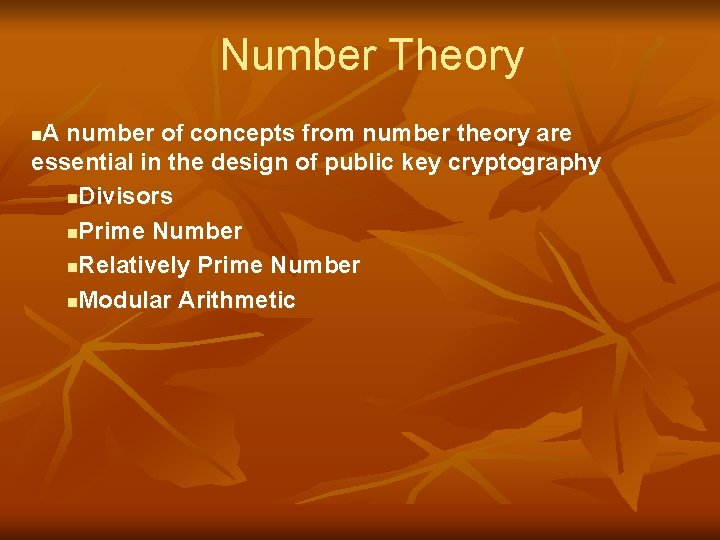Number Theory A number of concepts from number theory are essential in the design of public key cryptography n. Divisors n. Prime Number n. Relatively Prime Number n. Modular Arithmetic nDivisors We say that b =/ 0 divides a if a = mb for some m, where a, b, and m are integers n That is, b divides a if there is no remainder on division n The notation b|a is commonly used to mean b divides a n Also, if b|a, we say that d is a divisor of a n eg. The positive divisor of 24 are 1, 2, 3, 4, 6, 8, 12, and 24 n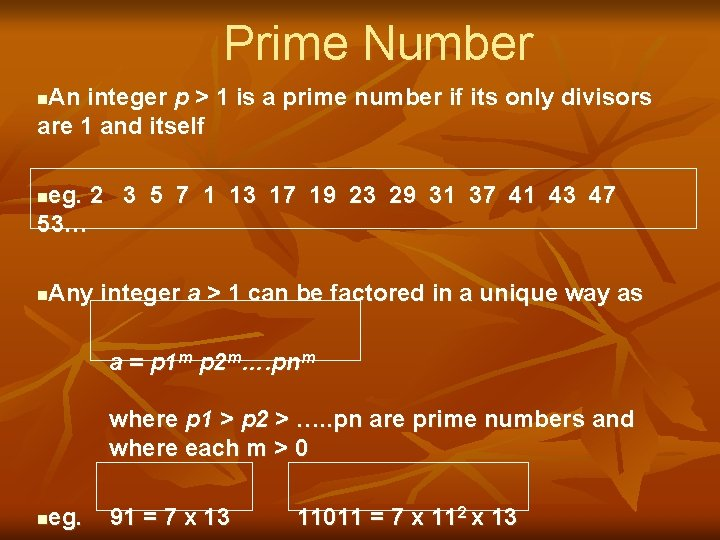Prime Number An integer p > 1 is a prime number if its only divisors are 1 and itself n eg. 2 3 5 7 1 13 17 19 23 29 31 37 41 43 47 53… n Any integer a > 1 can be factored in a unique way as n a = p 1 m p 2 m…. pnm where p 1 > p 2 > …. . pn are prime numbers and where each m > 0 eg. n 91 = 7 x 13 11011 = 7 x 112 x 13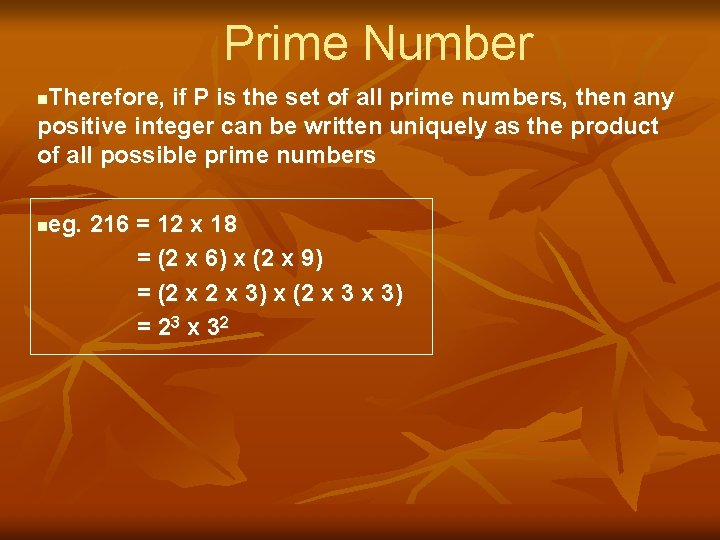Prime Number Therefore, if P is the set of all prime numbers, then any positive integer can be written uniquely as the product of all possible prime numbers n eg. 216 = 12 x 18 = (2 x 6) x (2 x 9) = (2 x 3) x (2 x 3) = 2 3 x 32 n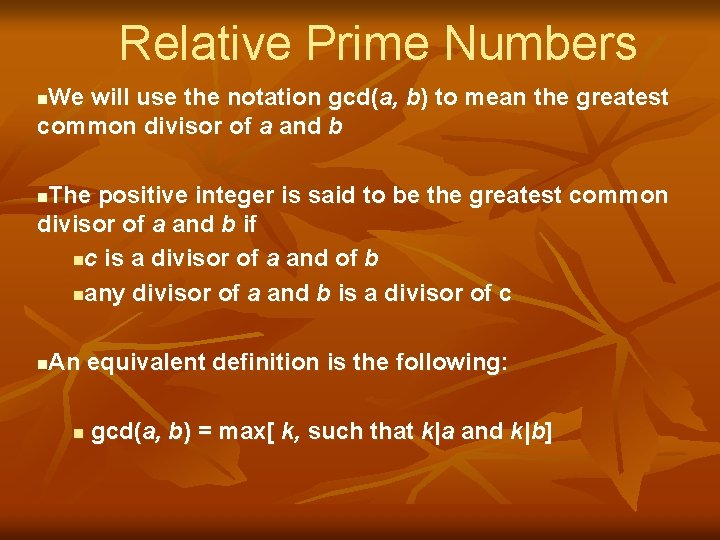Relative Prime Numbers We will use the notation gcd(a, b) to mean the greatest common divisor of a and b n The positive integer is said to be the greatest common divisor of a and b if nc is a divisor of a and of b nany divisor of a and b is a divisor of c n An equivalent definition is the following: n n gcd(a, b) = max[ k, such that k|a and k|b]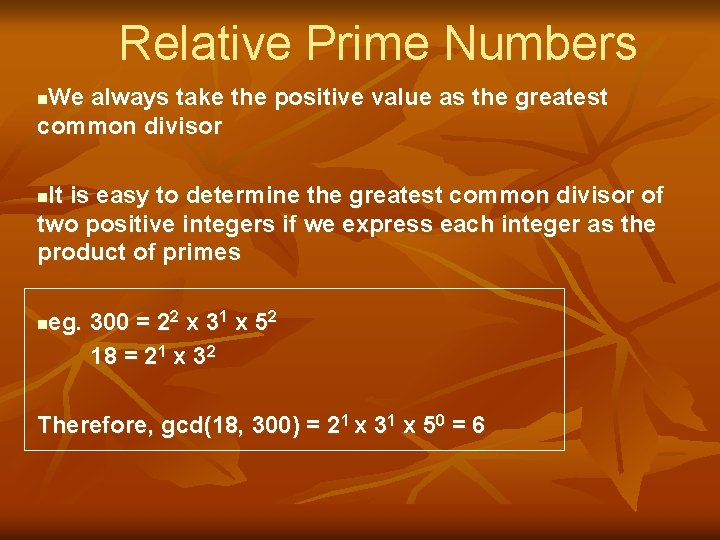Relative Prime Numbers We always take the positive value as the greatest common divisor n It is easy to determine the greatest common divisor of two positive integers if we express each integer as the product of primes n eg. 300 = 22 x 31 x 52 18 = 21 x 32 n Therefore, gcd(18, 300) = 21 x 31 x 50 = 6Relative Prime Numbers The integers a and b are relatively prime if they have no prime factors in common, that is, if their only common factors is 1. n This is equivalent to saying that a and b are relatively prime if gcd(a, b) = 1 n eg. 8 and 15 are relatively prime because the divisors of 8 are 1, 2, 4, and 8, and the divisors of 15 are 1, 3, 5, and 15. So 1 is the only number on both lists nModular Arithmetic Given any positive integer n and any integer a, we get a quotient q and a remainder r that obey the following relationship: n a = qn + r 0 < r < n; q = [a/n] where [x] is the largest integer less than or equal to x eg. a = 11; n = 7; 11 = 1 x 7 + 4; n Therefore we can say that r=4 11 = 1 x 7 + 4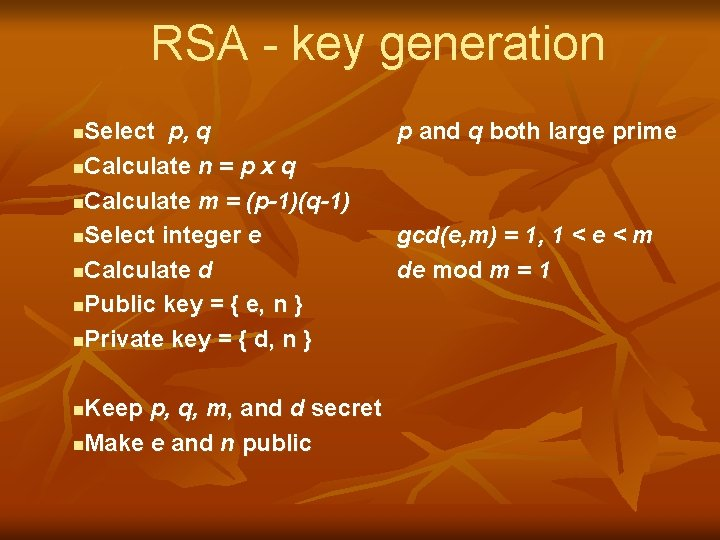RSA - key generation Select p, q n. Calculate n = p x q n. Calculate m = (p-1)(q-1) n. Select integer e n. Calculate d n. Public key = { e, n } n. Private key = { d, n } n Keep p, q, m, and d secret n. Make e and n public n p and q both large prime gcd(e, m) = 1, 1 < e < m de mod m = 1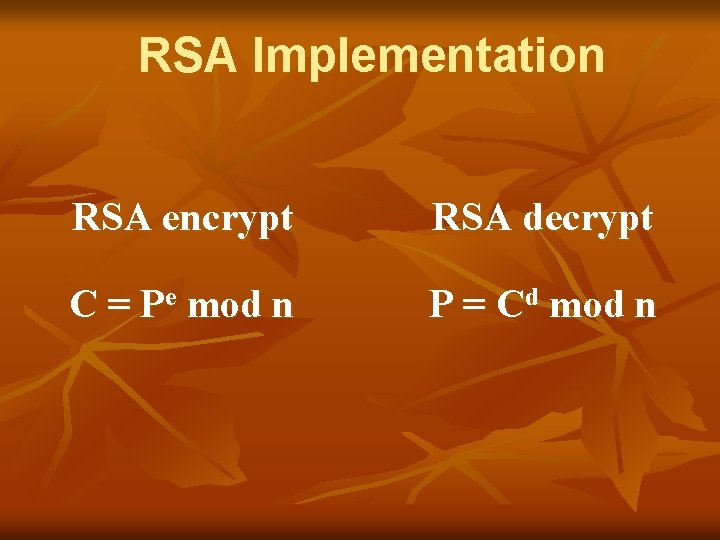RSA Implementation RSA encrypt RSA decrypt C = Pe mod n P = Cd mod n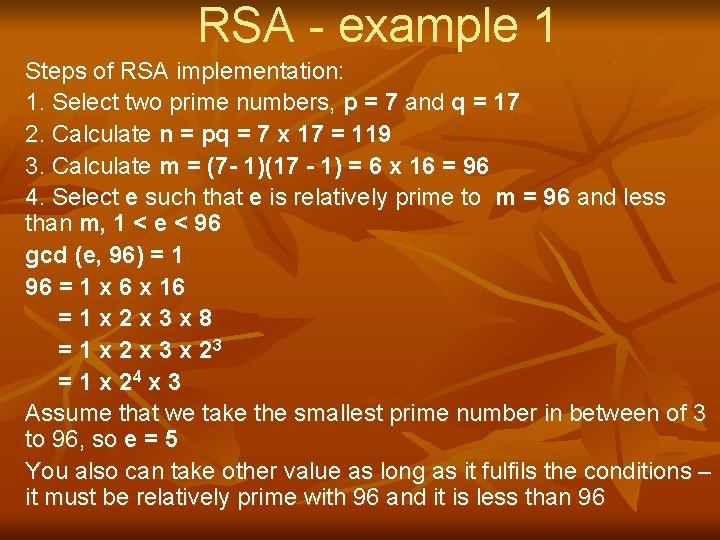RSA - example 1 Steps of RSA implementation: 1. Select two prime numbers, p = 7 and q = 17 2. Calculate n = pq = 7 x 17 = 119 3. Calculate m = (7 - 1)(17 - 1) = 6 x 16 = 96 4. Select e such that e is relatively prime to m = 96 and less than m, 1 < e < 96 gcd (e, 96) = 1 96 = 1 x 6 x 16 =1 x 2 x 3 x 8 = 1 x 2 x 3 x 23 = 1 x 24 x 3 Assume that we take the smallest prime number in between of 3 to 96, so e = 5 You also can take other value as long as it fulfils the conditions – it must be relatively prime with 96 and it is less than 96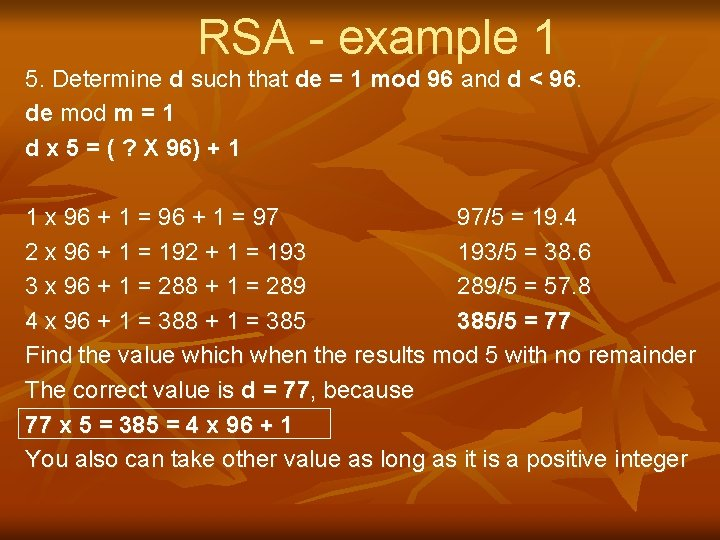RSA - example 1 5. Determine d such that de = 1 mod 96 and d < 96. de mod m = 1 d x 5 = ( ? X 96) + 1 1 x 96 + 1 = 97 97/5 = 19. 4 2 x 96 + 1 = 192 + 1 = 193/5 = 38. 6 3 x 96 + 1 = 288 + 1 = 289/5 = 57. 8 4 x 96 + 1 = 388 + 1 = 385/5 = 77 Find the value which when the results mod 5 with no remainder The correct value is d = 77, because 77 x 5 = 385 = 4 x 96 + 1 You also can take other value as long as it is a positive integerRSA - example 1 Therefore, n = 119, m = 96, e = 5, d = 77 The resulting keys are: Public key = { e, n } and Private key = { d, n} Public key = {5, 119} and Private key = {77, 119} Assume that P is the plaintext and C is the ciphertext The encryption is C = P 5 mod 119 The decryption is P = C 77 mod 119RSA - example 1 Public key = { e, n } and Private key = { d, n} Public key = {5, 119} and Private key = {77, 119} Given P = 4 The encryption is C = P 5 mod 119 C = 45 mod 119 = 1024 mod 119 = 72 The decryption is P = C 77 mod 119 P = 7227 mod 119 =4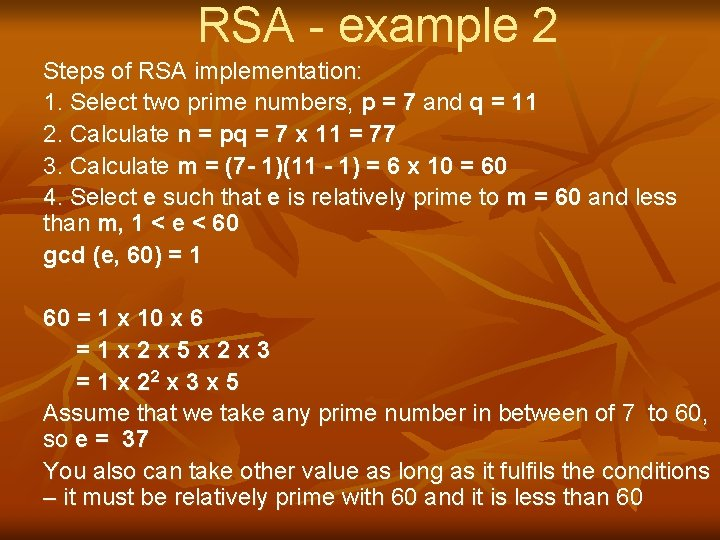RSA - example 2 Steps of RSA implementation: 1. Select two prime numbers, p = 7 and q = 11 2. Calculate n = pq = 7 x 11 = 77 3. Calculate m = (7 - 1)(11 - 1) = 6 x 10 = 60 4. Select e such that e is relatively prime to m = 60 and less than m, 1 < e < 60 gcd (e, 60) = 1 60 = 1 x 10 x 6 =1 x 2 x 5 x 2 x 3 = 1 x 22 x 3 x 5 Assume that we take any prime number in between of 7 to 60, so e = 37 You also can take other value as long as it fulfils the conditions – it must be relatively prime with 60 and it is less than 60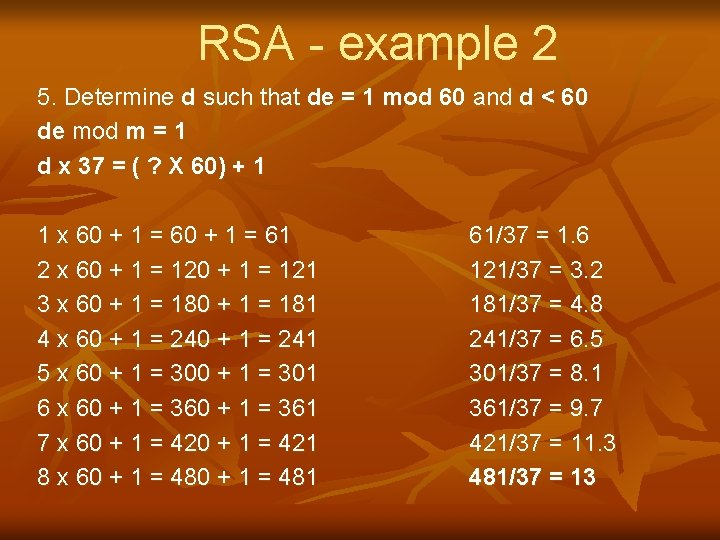RSA - example 2 5. Determine d such that de = 1 mod 60 and d < 60 de mod m = 1 d x 37 = ( ? X 60) + 1 1 x 60 + 1 = 61 2 x 60 + 1 = 121 3 x 60 + 1 = 181 4 x 60 + 1 = 241 5 x 60 + 1 = 301 6 x 60 + 1 = 361 7 x 60 + 1 = 421 8 x 60 + 1 = 481 61/37 = 1. 6 121/37 = 3. 2 181/37 = 4. 8 241/37 = 6. 5 301/37 = 8. 1 361/37 = 9. 7 421/37 = 11. 3 481/37 = 13RSA - example 2 Find the value which when the results mod 5 with no remainder The correct value is d = 13, because 13 x 37 = 481 = 8 x 60 + 1 You also can take other value as long as it is a positive integerRSA - example 2 Therefore, n = 77, m = 60, e = 37, d = 13 The resulting keys are: Public key = { e, n } and Private key = { d, n} Public key = {37, 77} and Private key = {13, 77} Assume that P is the plaintext and C is the ciphertext The encryption is C = P 37 mod 77 The decryption is P = C 13 mod 77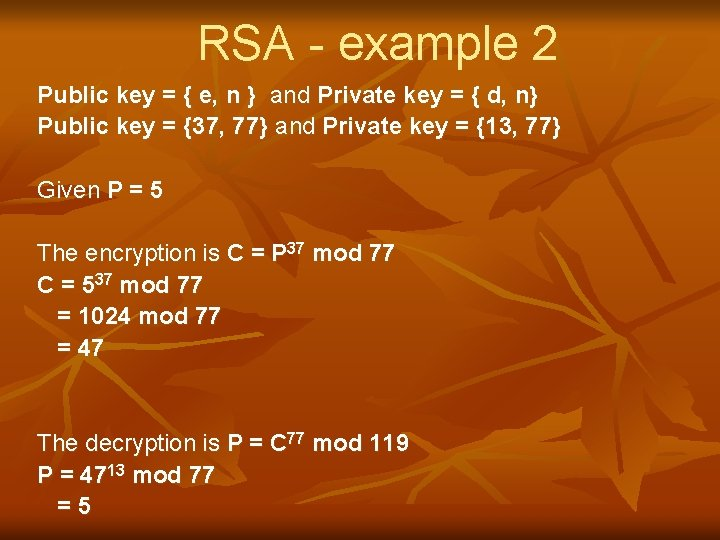RSA - example 2 Public key = { e, n } and Private key = { d, n} Public key = {37, 77} and Private key = {13, 77} Given P = 5 The encryption is C = P 37 mod 77 C = 537 mod 77 = 1024 mod 77 = 47 The decryption is P = C 77 mod 119 P = 4713 mod 77 =5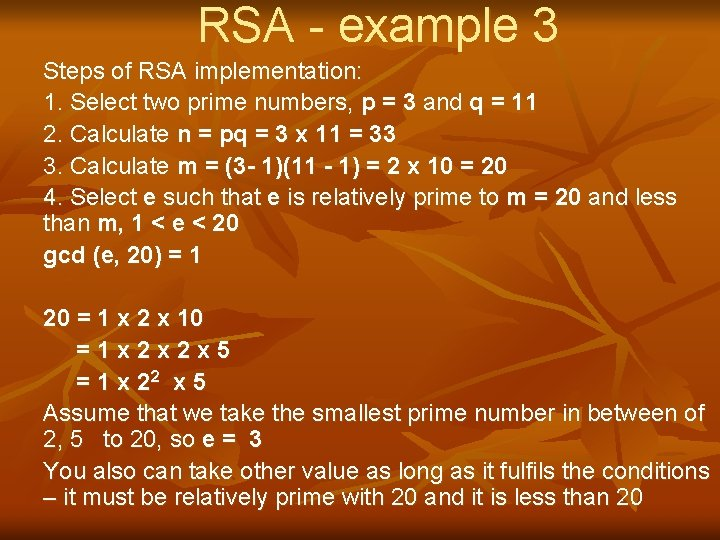RSA - example 3 Steps of RSA implementation: 1. Select two prime numbers, p = 3 and q = 11 2. Calculate n = pq = 3 x 11 = 33 3. Calculate m = (3 - 1)(11 - 1) = 2 x 10 = 20 4. Select e such that e is relatively prime to m = 20 and less than m, 1 < e < 20 gcd (e, 20) = 1 20 = 1 x 2 x 10 =1 x 2 x 2 x 5 = 1 x 22 x 5 Assume that we take the smallest prime number in between of 2, 5 to 20, so e = 3 You also can take other value as long as it fulfils the conditions – it must be relatively prime with 20 and it is less than 20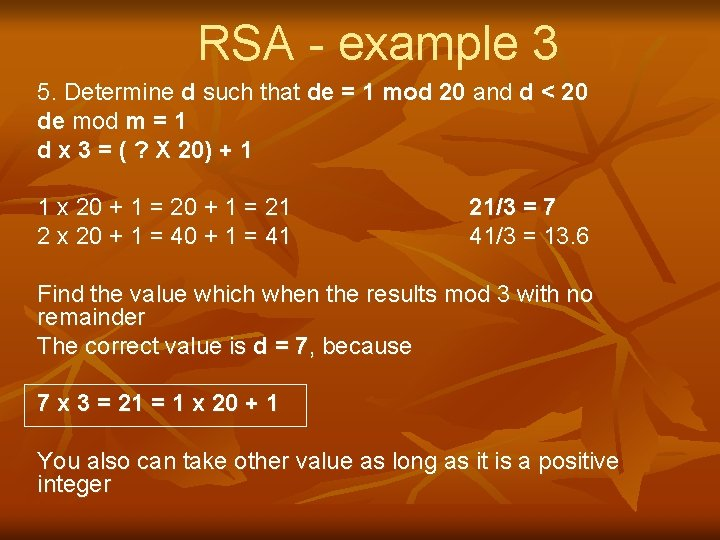RSA - example 3 5. Determine d such that de = 1 mod 20 and d < 20 de mod m = 1 d x 3 = ( ? X 20) + 1 1 x 20 + 1 = 21 2 x 20 + 1 = 41 21/3 = 7 41/3 = 13. 6 Find the value which when the results mod 3 with no remainder The correct value is d = 7, because 7 x 3 = 21 = 1 x 20 + 1 You also can take other value as long as it is a positive integerRSA - example 3 Therefore, n = 33, m = 20, e = 3, d = 7 The resulting keys are: Public key = { e, n } and Private key = { d, n} Public key = {3, 33} and Private key = {7, 33} Assume that P is the plaintext and C is the ciphertext The encryption is C = P 3 mod 33 The decryption is P = C 7 mod 33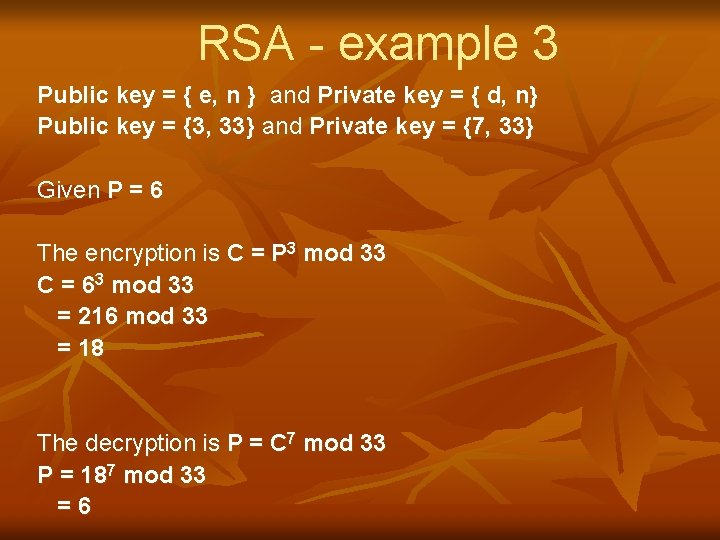RSA - example 3 Public key = { e, n } and Private key = { d, n} Public key = {3, 33} and Private key = {7, 33} Given P = 6 The encryption is C = P 3 mod 33 C = 63 mod 33 = 216 mod 33 = 18 The decryption is P = C 7 mod 33 P = 187 mod 33 =6RSA - example 4 Steps of RSA implementation: 1. Select two prime numbers, p = 5 and q = 11 2. Calculate n = pq = 5 x 11 = 55 3. Calculate m = (5 - 1)(11 - 1) = 4 x 10 = 40 4. Select e such that e is relatively prime to m = 40 and less than m, 1 < e < 40 gcd (e, 40) = 1 40 = 1 x 4 x 10 =1 x 2 x 2 x 2 x 5 = 1 x 23 x 5 Assume that we take the smallest prime number in between of 2, 5 to 40, so e = 3 You also can take other value as long as it fulfils the conditions – it must be relatively prime with 40 and it is less than 40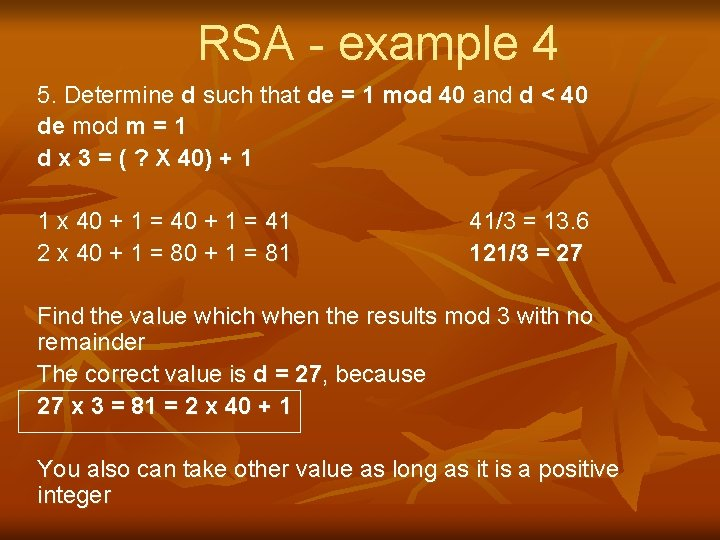RSA - example 4 5. Determine d such that de = 1 mod 40 and d < 40 de mod m = 1 d x 3 = ( ? X 40) + 1 1 x 40 + 1 = 41 2 x 40 + 1 = 81 41/3 = 13. 6 121/3 = 27 Find the value which when the results mod 3 with no remainder The correct value is d = 27, because 27 x 3 = 81 = 2 x 40 + 1 You also can take other value as long as it is a positive integerRSA - example 4 Therefore, n = 55, m = 40, e = 3, d = 27 The resulting keys are: Public key = { e, n } and Private key = { d, n} Public key = {3, 55} and Private key = {27, 55} Assume that P is the plaintext and C is the ciphertext The encryption is C = P 3 mod 55 The decryption is P = C 27 mod 55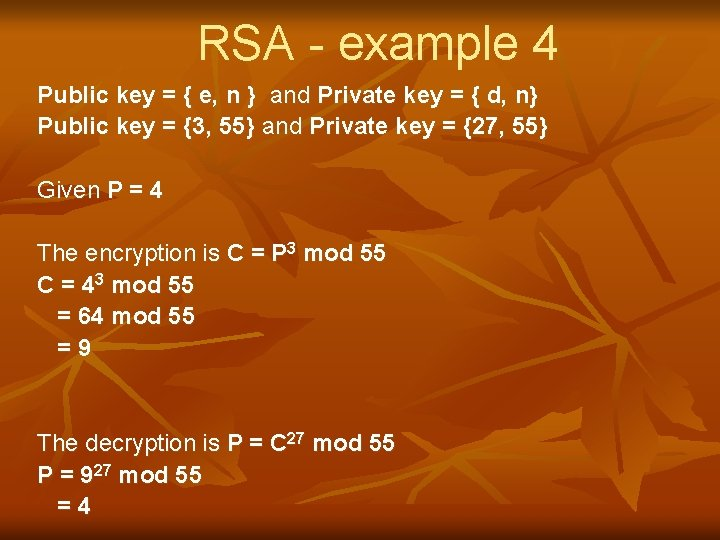RSA - example 4 Public key = { e, n } and Private key = { d, n} Public key = {3, 55} and Private key = {27, 55} Given P = 4 The encryption is C = P 3 mod 55 C = 43 mod 55 = 64 mod 55 =9 The decryption is P = C 27 mod 55 P = 927 mod 55 =4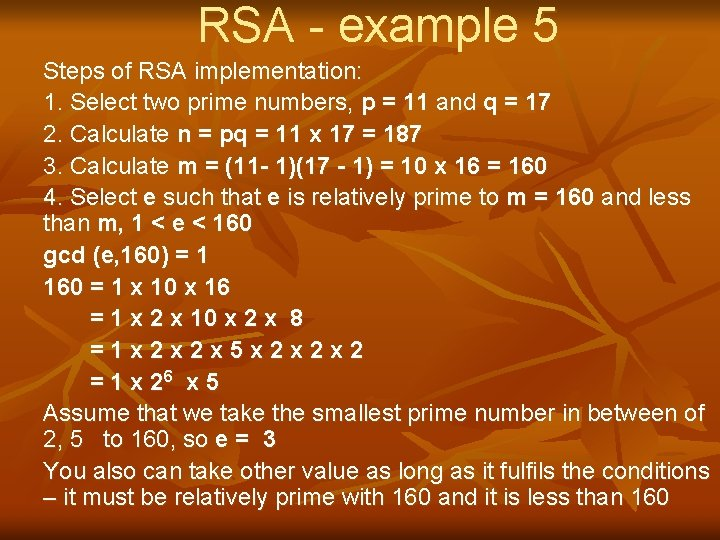RSA - example 5 Steps of RSA implementation: 1. Select two prime numbers, p = 11 and q = 17 2. Calculate n = pq = 11 x 17 = 187 3. Calculate m = (11 - 1)(17 - 1) = 10 x 16 = 160 4. Select e such that e is relatively prime to m = 160 and less than m, 1 < e < 160 gcd (e, 160) = 1 160 = 1 x 10 x 16 = 1 x 2 x 10 x 2 x 8 =1 x 2 x 2 x 5 x 2 x 2 x 2 = 1 x 26 x 5 Assume that we take the smallest prime number in between of 2, 5 to 160, so e = 3 You also can take other value as long as it fulfils the conditions – it must be relatively prime with 160 and it is less than 160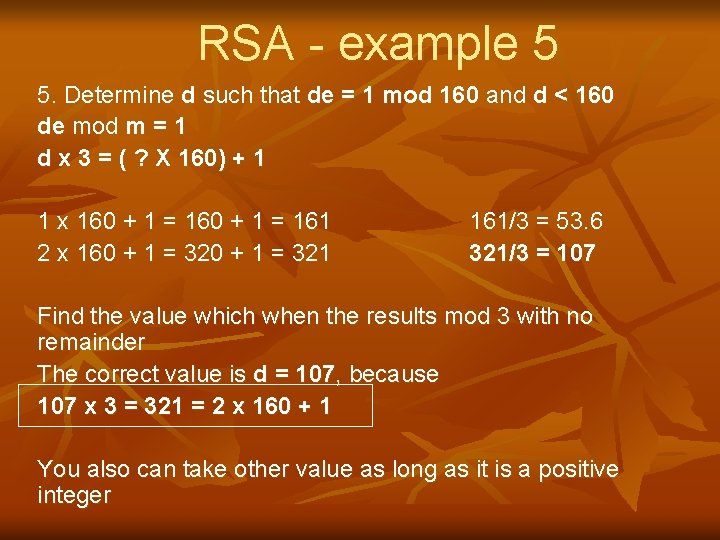RSA - example 5 5. Determine d such that de = 1 mod 160 and d < 160 de mod m = 1 d x 3 = ( ? X 160) + 1 1 x 160 + 1 = 161 2 x 160 + 1 = 321 161/3 = 53. 6 321/3 = 107 Find the value which when the results mod 3 with no remainder The correct value is d = 107, because 107 x 3 = 321 = 2 x 160 + 1 You also can take other value as long as it is a positive integerRSA - example 5 Therefore, n = 187, m = 160, e = 3, d = 107 The resulting keys are: Public key = { e, n } and Private key = { d, n} Public key = {3, 187} and Private key = {107, 187} Assume that P is the plaintext and C is the ciphertext The encryption is C = P 3 mod 187 The decryption is P = C 107 mod 187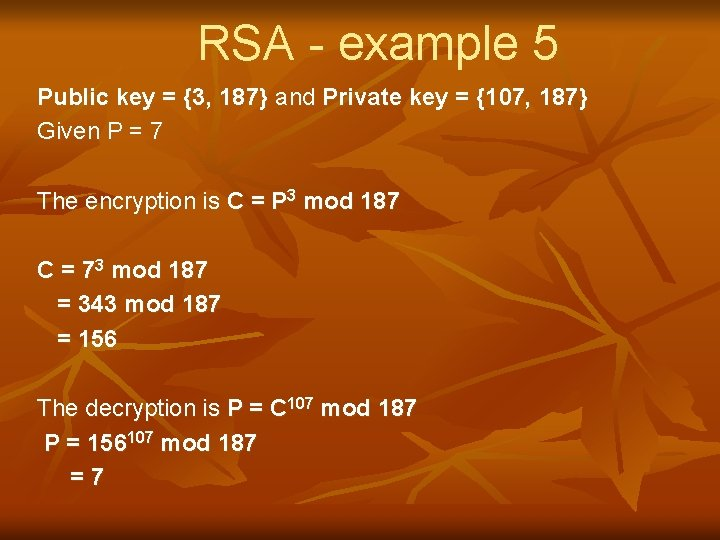RSA - example 5 Public key = {3, 187} and Private key = {107, 187} Given P = 7 The encryption is C = P 3 mod 187 C = 73 mod 187 = 343 mod 187 = 156 The decryption is P = C 107 mod 187 P = 156107 mod 187 =7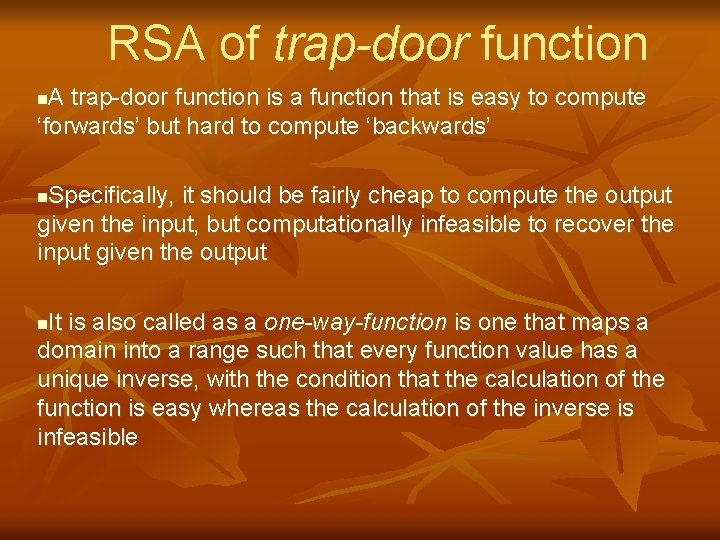RSA of trap-door function A trap-door function is a function that is easy to compute ‘forwards’ but hard to compute ‘backwards’ n Specifically, it should be fairly cheap to compute the output given the input, but computationally infeasible to recover the input given the output n It is also called as a one-way-function is one that maps a domain into a range such that every function value has a unique inverse, with the condition that the calculation of the function is easy whereas the calculation of the inverse is infeasible n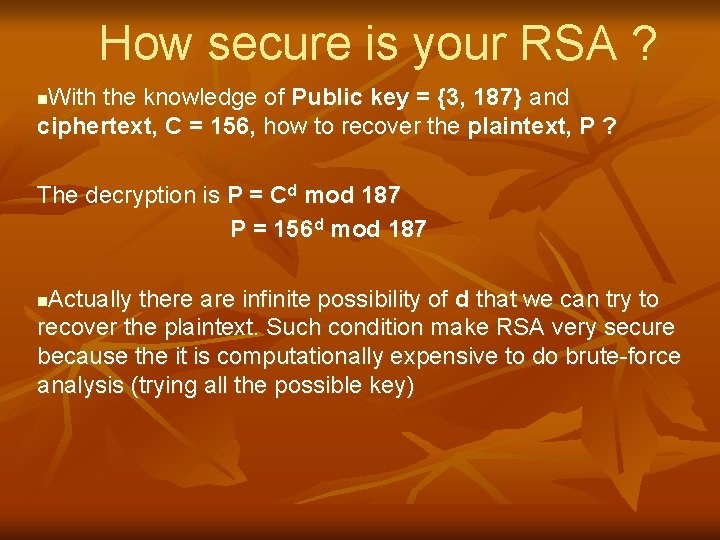How secure is your RSA ? With the knowledge of Public key = {3, 187} and ciphertext, C = 156, how to recover the plaintext, P ? n The decryption is P = Cd mod 187 P = 156 d mod 187 Actually there are infinite possibility of d that we can try to recover the plaintext. Such condition make RSA very secure because the it is computationally expensive to do brute-force analysis (trying all the possible key) nHow secure is your RSA ? In 1977, RSA inventors offered a \$100 reward for the return of a plaintext sentences, an event they predicted might not occur for some 40 quadrillion years with the public key length of 129 decimal digits or around 428 bits n In April 1994, a group working over the Internet claimed the prize after only eight months of work n